# Linear Prediction for Spatial and Spatio-Temporal Fields

25 September 2018

$\newcommand{\Expect}{\mathbb{E}\left[ #1 \right]} \newcommand{\Var}{\mathrm{Var}\left[ #1 \right]} \newcommand{\SampleVar}{\widehat{\mathrm{Var}}\left[ #1 \right]} \newcommand{\Cov}{\mathrm{Cov}\left[ #1 \right]} \newcommand{\TrueRegFunc}{\mu} \newcommand{\EstRegFunc}{\widehat{\TrueRegFunc}} \DeclareMathOperator{\tr}{tr} \DeclareMathOperator*{\argmin}{argmin} \DeclareMathOperator{\det}{det} \newcommand{\TrueNoise}{\epsilon} \newcommand{\EstNoise}{\widehat{\TrueNoise}}$

# In our previous episode

• General approach to optimal linear prediction
• Predict $$Y$$ from $$\vec{Z} = [Z_1, Z_2, \ldots Z_p]$$
• Prediction is $$\alpha + \vec{\beta} \cdot \vec{Z}$$
• Best $$\alpha = \Expect{Y} - \vec{\beta} \cdot \Expect{\vec{Z}}$$
• Best $$\beta = \Var{\vec{Z}}^{-1} \Cov{\vec{Z}, Y}$$
• Today: application to spatial and spatio-temporal data
• What can this do for us?
• How do we find the covariances?

# Optimal linear prediction for spatial data

• Given: $$X(r_1), X(r_2), \ldots X(r_n)$$
• Desired: prediction of $$X(r_0)$$
$\begin{eqnarray} \EstRegFunc(r_0) & = & \alpha + \vec{\beta} \cdot \left[\begin{array}{c} X(r_1) \\ X(r_2) \\ \vdots \\ X(r_n) \end{array}\right]\\ \alpha & = & \Expect{X(r_0)} - \vec{\beta} \cdot \left[\begin{array}{c} \Expect{X(r_1)}\\ \Expect{X(r_2)} \\ \vdots \\ \Expect{X(r_n)}\end{array}\right] ~ \text{(goes away if everything's centered)}\\ \vec{\beta} & = & {\left[\begin{array}{cccc} \Var{X(r_1)} & \Cov{X(r_1), X(r_2)} & \ldots & \Cov{X(r_1), X(r_n)}\\ \Cov{X(r_1), X(r_2)} & \Var{X(r_2)} & \ldots & \Cov{X(r_2), X(r_n)}\\ \vdots & \vdots & \ldots & \vdots\\ \Cov{X(r_1), X(r_n)} & \Cov{X(r_2), X(r_n)} & \ldots & \Var{X(r_n)}\end{array}\right]}^{-1} \left[\begin{array}{c} \Cov{X(r_0), X(r_1)}\\ \Cov{X(r_0), X(r_2)}\\ \vdots \\ \Cov{X(r_0), X(r_n)}\end{array}\right] \end{eqnarray}$

# This should look familiar

• It’s exactly the same as optimal linear prediction for time series
• Independently re-invented in geology by D. G. Krige in 1951
• Mining engineer who wanted to estimate mineral value of new sites from old ones
• Refined by French geostatisticians in the 1960s
• “kriging” = French mathematicians inventing an English verb for “using optimal linear prediction on spatial data”
• Reference: Krige (1981)

# What do we need to know?

• $$\Expect{X(r)}$$
• $$\Var{X(r)}$$
• $$\Cov{X(r), X(q)}$$
• Problem: we only have one observation of each $$X(r)$$

# Expectation values

• We can estimate $$\Expect{X(r)}$$ by smoothing
• Assuming $$\TrueRegFunc(r)$$ doesn’t change very rapidly
• Need to pick a smoother
• Assuming $$\Expect{X(r)} =$$ constant is one choice of smoother…
• Moving averages can work, splines are nice…

# What about variance and covariance?

$\Cov{X(r), X(q)} = \Expect{X(r)X(q)} -\TrueRegFunc(r) \TrueRegFunc(q) = \Expect{(X(r)-\TrueRegFunc(r))(X(q) - \TrueRegFunc(q))}$

• If we saw $$n$$ realizations of $$X(r)$$ and $$X(q)$$, we could use $\Cov{X(r), X(q)} \approx \frac{1}{n}\sum_{i=1}^{n}{(X^{(i)}(r)-\TrueRegFunc(r))(X^{(i)}(q) - \TrueRegFunc(q))}$

• but we see $$X(r)$$ and $$X(q)$$ only once

• To get anywhere, we need to assume something about how the covariance function works
• Useful assumptions are restrictions on $$\Cov{X(r), X(q)}$$
• We could assume some covariances are exactly equal, so we can pool the corresponding pairs of points in estimating the covariance
• Or we could assume $$\Cov{X(r), X(q)}$$ is a smooth function of $$r$$ and $$q$$, and use smoothing
• Or both

# Some restrictions on $$\Cov{X(r), X(q)}$$

• Stationarity: for any displacement $$h$$, $\Cov{X(r), X(r+h)} = \Cov{X(q), X(q+h)} \equiv \gamma(h)$
• Often also assume $$\TrueRegFunc(q) =$$ constant
• Lets us pool pairs of points separated by the same displacement
• Can be checked:
• Sub-divide data in space
• Re-estimate the covariance function in each spatial region
• Do they match?

# Some restrictions on $$\Cov{X(r), X(q)}$$

• Isotropy (in 2 or 3D): if $$\|h\| = \|k\|$$, then $\Cov{X(r), X(r+h)} = \Cov{X(r), X(r+k)}$
• Lets us pool pairs of points separated by the same distance from $$r$$
• Can also be stationary and isotropic
• That lets us pool pairs of points at the same distance
• Can be checked:
• Sub-divide data into pairs of points separated in the same (or similar) directions
• Re-estimate the covariance function along each direction
• Do they match?

# Some restrictions on $$\Cov{X(r), X(q)}$$

• Separability: In 2D, $$r=(r_1, r_2)$$ and $$q=(q_1, q_2)$$, $\Cov{X(r), X(q)} = C_1(r_1, q_1) C_2(r_2, q_2)$
• Similarly for 3D
• Lets us pool pairs of points with same $$r_1, q_1$$ (for $$C_1$$)
• Can also be stationary and separable
• Lets us pool pairs of points with same $$r_1 - q_1$$ (for $$C_1$$)
• Often unphysical but assumed for statistical convenience
• Is separable and isotropic possible?
• Can be checked:
• How?

# Estimating a stationary covariance function

• For each $$h$$, $$n_h =$$ number of $$r_i, r_j$$ pairs with $$r_i - r_j = h$$ $\hat{\gamma}(h) = \frac{1}{n_h} \sum_{i, j ~ : ~ r_i - r_j = h}{(X(r_i) - \EstRegFunc(r_i)) (X(r_j) - \EstRegFunc(r_j))}$
• Works best on a regular grid…
• … which we don’t always have

# Think of the Irish wind data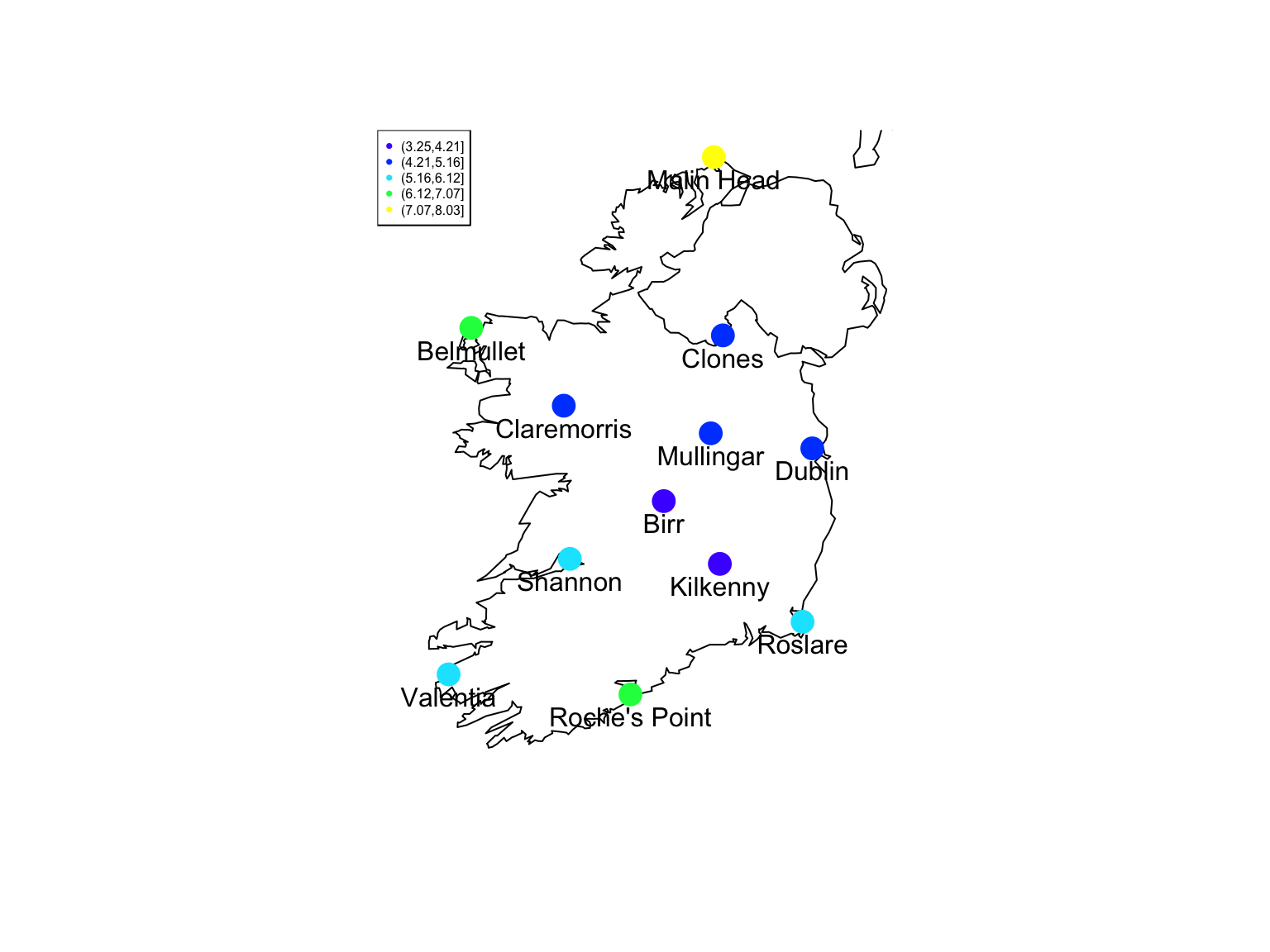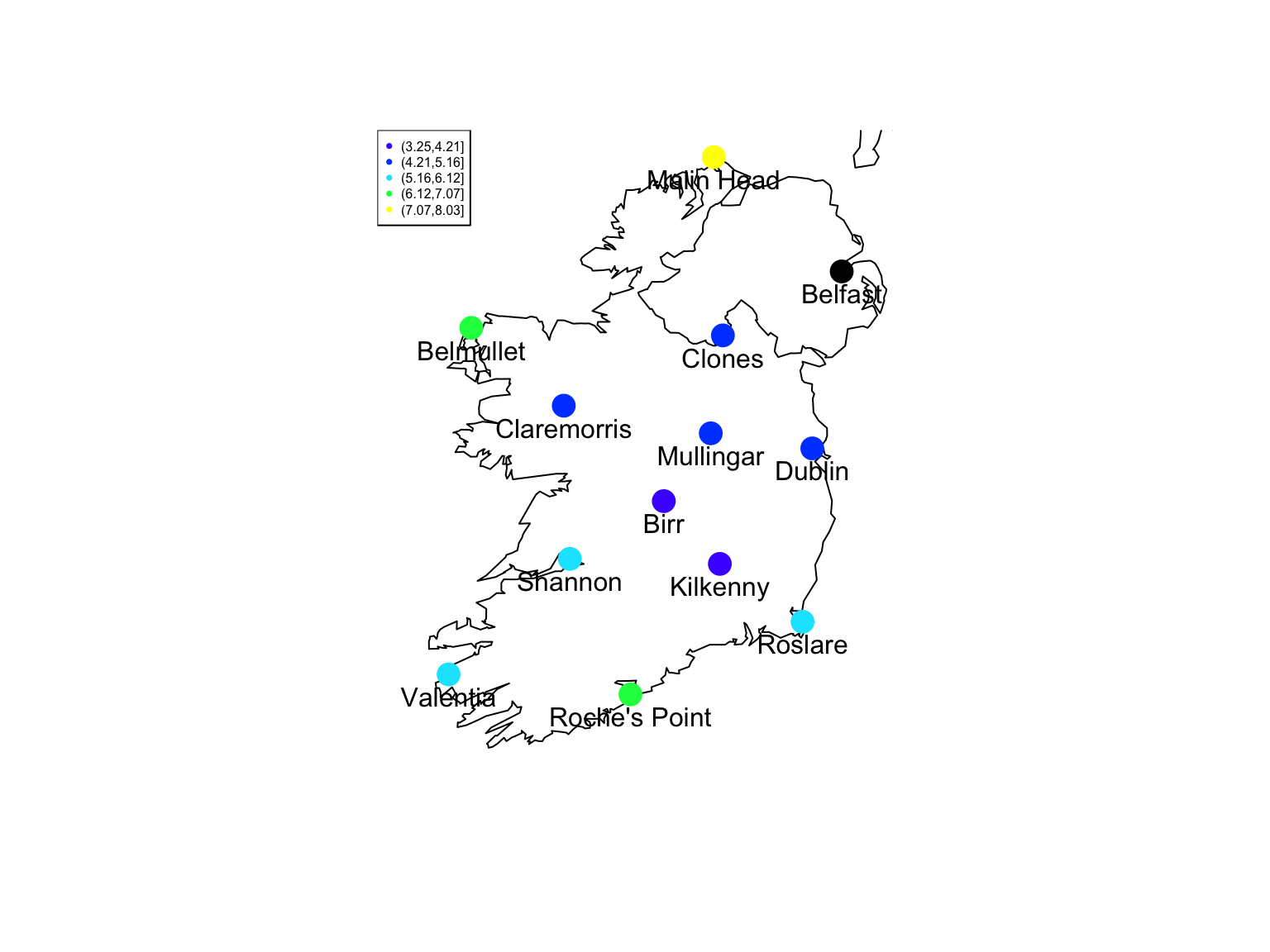# Try a stationary, isotropic covariance

• Assume $$\TrueRegFunc =$$ constant so $$\EstRegFunc= 5.26$$.
• Assume $$\Cov{X(r), X(q)} = \gamma(\|r-q\|)$$
• Plot $$(X(r_i) - \EstRegFunc(r_i)) (X(r_j) - \EstRegFunc(r_j))$$ against $$r_i - r_j$$
global.mean <- mean(wind.loc$MeanWind) distances <- spDists(coordinates(wind.loc), longlat = TRUE) c.rq <- outer(wind.loc$MeanWind - global.mean, wind.loc$MeanWind - global.mean, "*") rho.rq <- c.rq/var(wind.loc$MeanWind)

# Try a stationary, isotropic covariance

plot(x = as.vector(distances), y = as.vector(rho.rq), xlab = "Distance (km)",
ylab = expression((x(r) - bar(x))(x(q) - bar(x))/sigma^2))
lines(smooth.spline(x = as.vector(distances), y = as.vector(rho.rq), cv = TRUE))
L.rough <- -log(0.14)/100
curve(exp(-L.rough * x), add = TRUE, col = "blue")
legend("topright", legend = c("Data", "Spline", "Exponential"), pch = c(1, NA,
NA), lty = c("blank", "solid", "solid"), col = c("black", "black", "blue"))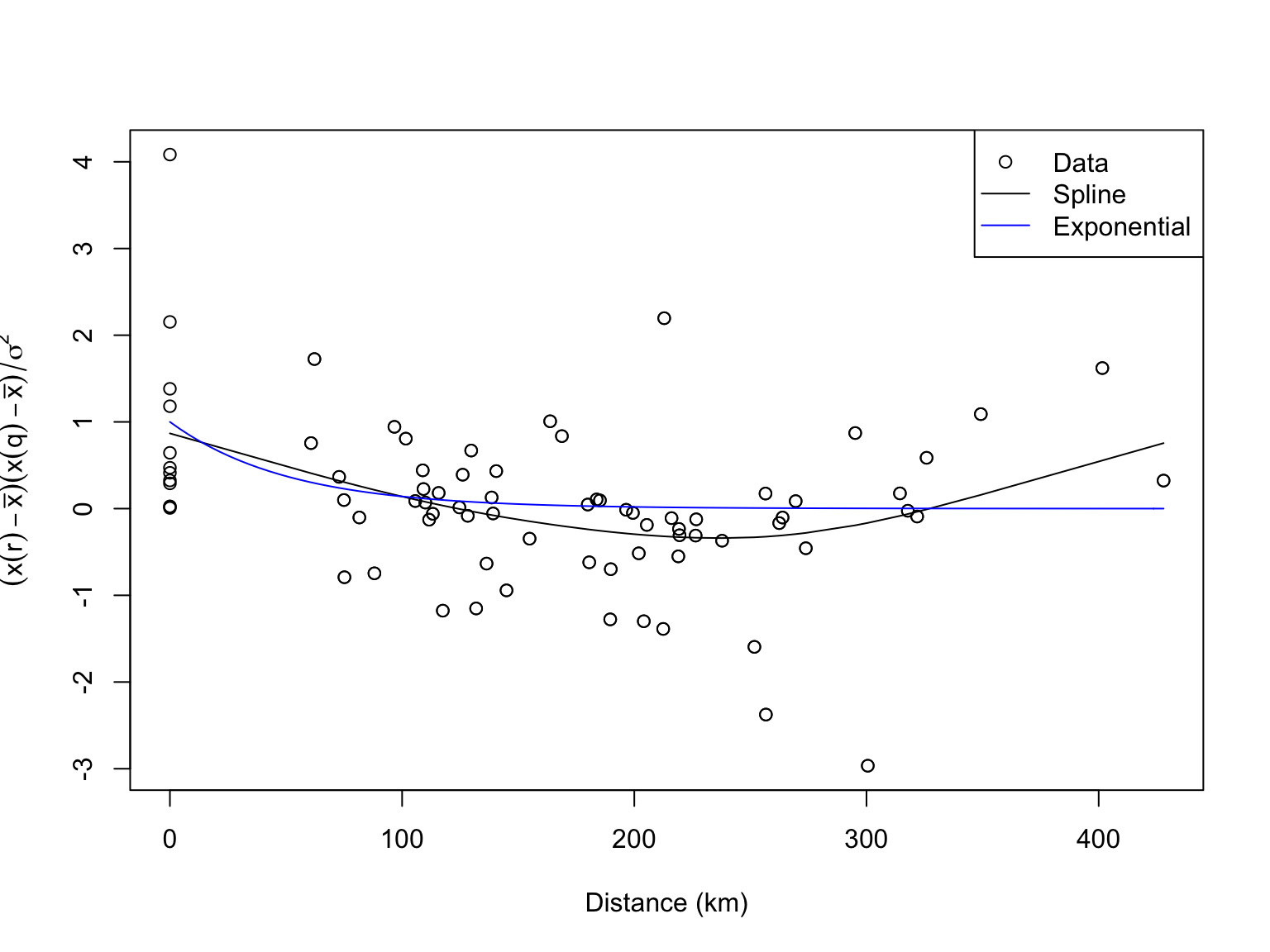# Try a stationary, isotropic covariance

• Fit the spline first to guide the eye
• Tried exponential decay because that often works
• $$\rho(\|h\|) = e^{-\|h\| \lambda}$$, $$1/\lambda=$$ correlation length
• Rough guess at $$\lambda$$ from how much the spline had fallen in 100km
• In this case, $$1/\lambda \approx 51 \mathrm{km}$$
• Could improve this through fitting the exponential model (see back-up slides on nonlinear least squares)
• Sometimes try $$\rho(\|h\|) = c\mathbf{1}(h=0) + (1-c)e^{-\|h\| \lambda}$$, nugget effect

# Kriging

wind.prediction <- function(r, L) {
fit <- c(fit = NA, se = NA)
global.mean <- mean(wind.loc$MeanWind) global.var <- var(wind.loc$MeanWind)
distances.to.r <- spDistsN1(pts = coordinates(wind.loc), pt = r, longlat = TRUE)
distances.among.q <- spDists(coordinates(wind.loc), longlat = TRUE)
corYZ <- exp(-L * distances.to.r)
corZ <- exp(-L * distances.among.q)
Z <- wind.loc$MeanWind fit["fit"] <- global.mean + (Z - global.mean) %*% solve(corZ) %*% corYZ fit["se"] <- sqrt(global.var - t(corYZ) %*% solve(corZ) %*% corYZ) return(fit) } wind.prediction(r = as.numeric(belfast.longlat), L = L.rough) ## fit se ## 5.362427 1.358965 # Spatio-temporal prediction • Once more, with feeling • The math is exactly the same • Again: all about estimating covariance functions • Stationarity: over space? over time? both? • If over both, $$\Cov{X(r,t), X(q,s)} = \Cov{X(0,0), X(r-q, s-t)} = \gamma(r-q, s-t)$$ • Taylor hypothesis (Gneiting, Genton, and Guttorp 2007): for some velocity $$v$$, $$\gamma(0,t) = \gamma(vt,0)$$ • (after G. I. Taylor, see Batchelor (1996); sometimes useful in fluid flows) • Isotropy: usually only makes sense for space, not for space+time • $$\|h\| = \|k\|$$ $$\Rightarrow$$ $$\Cov{X(r,t), X(r+h, s)} = \Cov{X(q,t), X(q+k, s)}$$ • Separability: space from time? coordinates of space? • Space-time separability is often even more artificial than spatial separability # Summary • Spatial and spatio-temporal prediction works just like time series • The only trick is estimating covariances # Backup: Some figures from Krige (1981)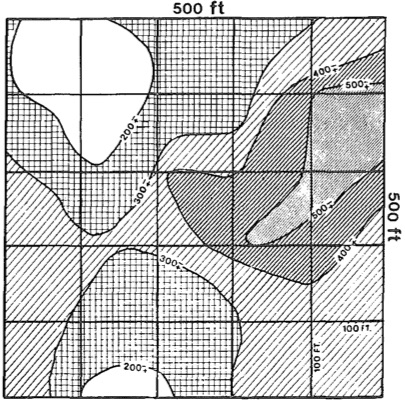“Typical gold inch-dwt [=concentration] trend surfaces in the Klerksdorp goldfield on the basis of two-dimensional moving averages [= kriging]… Moving averages of $$100 \times 100$$ ft areas within a mined-out section of $$500\times 500$$ ft” (p. 28, caption of fig. 21a) # Backup: Some figures from Krige (1981)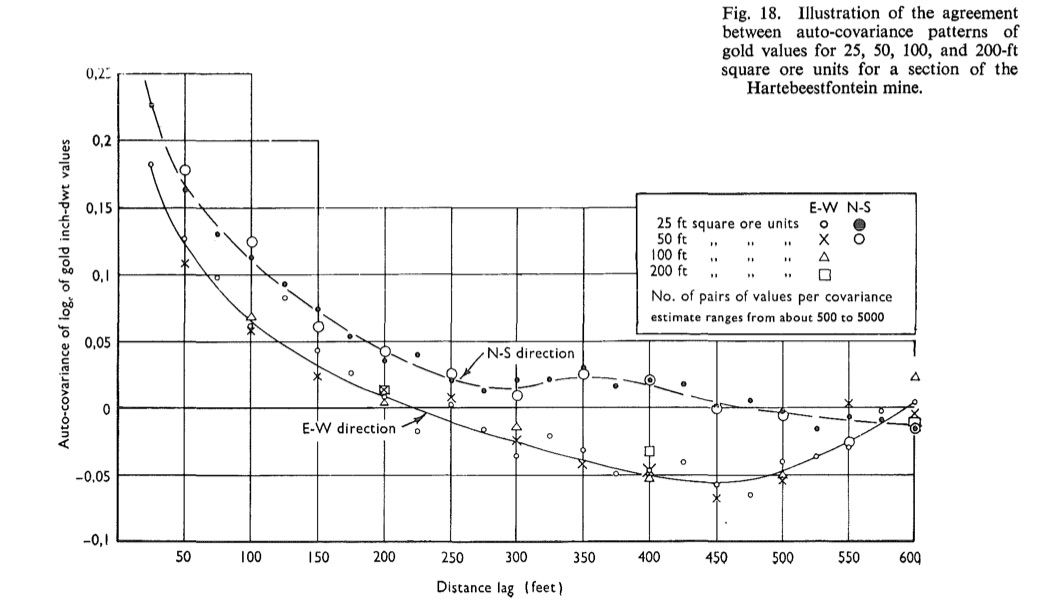“Illustration of the agreement between auto-covariance patterns of gold values for 25, 50, 100, and 200-ft square ore units for a section of the Hartebeestfontein mine” (p. 23, caption of fig. 18) # Backup: Valid covariance functions • $$\Cov{X(r), X(q)}$$ can’t be just any function of $$r$$ and $$q$$ • $$\Cov{X(r), X(r)} \geq 0$$, all implied correlations $$\in [-1, 1]$$, etc. • For every valid $$\Cov{X(r), X(q)}$$, for any set of points $$r_1, r_2, \ldots r_n$$, the matrix $$c_{ij} = \Cov{X(r_i), X(r_j)}$$ is non-negative definite • i.e., $$\mathbf{v}^T \mathbf{c} \mathbf{v} \geq 0$$ for any vector $$\mathbf{v}$$ • Any function of $$r$$ and $$q$$ which always leads to non-negative definite matrices is the covariance function of some distribution • The point of the French mathematicians’ work on geostatistics in the 1960s and 1970s was to realize this, and to use it to restrict the search for covariance functions to ones which are non-negative definite • Gneiting, Genton, and Guttorp (2007) has a good discussion, but needs Fourier analysis (next week!) # Backup: Parametric covariance (or correlation) estimation • $$\Cov{X(r), X(q)} = \Expect{(X(r)-\TrueRegFunc(r))(X(q)-\TrueRegFunc(q))}$$ • For each pair of points $$r$$ and $$q$$, create the product of deviations $$C(r,q) = (X(r) - \EstRegFunc(r))(X(q) - \EstRegFunc(q))$$ • If we had many realizations of $$X(r), X(q)$$, we could average $$C(r,q)$$ to estimate $$\Cov{X(r), X(q)}$$ • Assume instead that we have a parametric form for the covariance, $$\Cov{X(r), X(q)} = f(r,q,\theta)$$ (parameter $$\theta$$ unknown) • E.g., $$f(r,q,\lambda, \sigma^2) = \sigma^2 e^{-\|r-q\|\lambda}$$ for a stationary, isotropic, exponentially-decaying covariance, with correlation length $$1/\lambda$$ and variance $$\sigma^2$$ • Then $$C(r,q) = f(r,q,\theta)+$$ noise, with noise having mean 0 • Use nonlinear least squares (NLS): $\hat{\theta} = \argmin_{\theta}{\frac{1}{n^2}\sum_{i=1}^{n}{\sum_{j=1}^{n}{{\left( C(r_i, r_j) - f(r_i, r_j, \theta)\right)}^2}}}$ • Can apply this to correlations rather than covariances if the variances are known, or assumed constant # Backup: Nonlinear least squares • General setting: $$Y=f(Z,\theta) +$$ noise • data $$(z_1, y_1), (z_2, y_2), \ldots (z_m, y_m)$$ • NLS estimate is $\hat{\theta} = \argmin_{\theta}{\frac{1}{m}{\sum_{i=1}^{m}{{\left( y_i - f(z_i, \theta)\right)}^2}}}$ • Statistical properties very similar to ordinary least squares, but computationally more annoying • Statistically, optimization methods are all pretty similar, and we’ll see the theory in a few weeks • Computationally, no closed-form solution (unlike OLS), so you need to iteratively approach the optimum • In R: either nls (specifically for nonlinear least squares) or optim (for general-purpose optimization) • Usually needs an initial guess about $$\theta$$ # Backup: Nonlinear least squares illustrated Using optim: rho.mse <- function(L) { mean((rho.rq - exp(-L * distances))^2) } L.optim <- optim(par = L.rough, fn = rho.mse, method = "BFGS") L.optim$par
##  0.02432098

so estimated correlation length is $$41 \mathrm{km}$$ rather than initial guess of $$51 \mathrm{km}$$

# Backup: Nonlinear least squares illustrated

Using nls:

nls(as.vector(rho.rq) ~ exp(-L * as.vector(distances)), start = list(L = L.rough))
## Nonlinear regression model
##   model: as.vector(rho.rq) ~ exp(-L * as.vector(distances))
##    data: parent.frame()
##       L
## 0.02428
##  residual sum-of-squares: 109.6
##
## Number of iterations to convergence: 5
## Achieved convergence tolerance: 3.649e-06